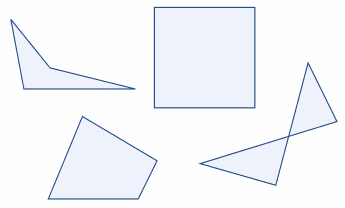(quad means four, lateral means side).

Any four-sided shape is a Quadrilateral.

But the sides have to be straight, and it has to be2-dimensional.

## Properties

• Four sides (edges)
• Four vertices (corners)
• The interior angles add up to 360 degrees: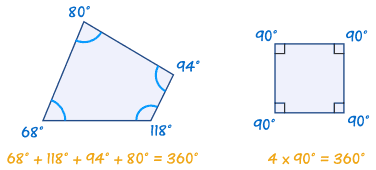Try drawing a quadrilateral, and measure the angles. They should add to 360°

There are special types of quadrilateral: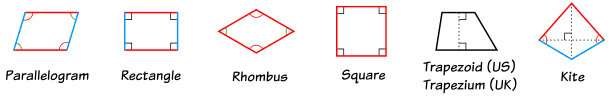Some types are also included in the definition of other types! For example a squarerhombus andrectangle are also parallelogramsSee below for more details.

Let us look at each type in turn:

## The Rectangle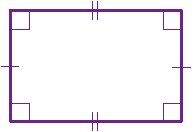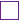means "right angle"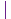andshow equal sides

rectangle is a four-sided shape where every angle is a right angle (90°).

Also opposite sides are parallel and of equal length.

## The Rhombus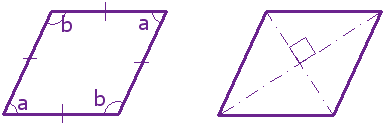rhombus is a four-sided shape where all sides have equal length.

Also opposite sides are parallel and opposite angles are equal.

Another interesting thing is that the diagonals (dashed lines in second figure) meet in the middle at a right angle. In other words they "bisect" (cut in half) each other at right angles.

A rhombus is sometimes called a rhomb or a diamond.

## The Square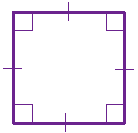means "right angle"show equal sides

square has equal sides and every angle is a right angle (90°)

Also opposite sides are parallel.

A square also fits the definition of a rectangle (all angles are 90°), and a rhombus (all sides are equal length).

## The Parallelogram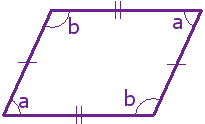parallelogram has opposite sides parallel and equal in length. Also opposite angles are equal (angles "a" are the same, and angles "b" are the same).

NOTE: Squares, Rectangles and Rhombuses are all Parallelograms!

### Example:parallelogram with:

• all sides equal and
• angles "a" and "b" as right angles

is a square!

## The Trapezoid (UK: Trapezium)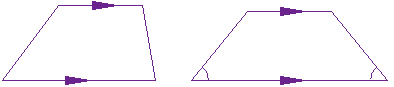Trapezoid Isosceles Trapezoid

trapezoid (called a trapezium in the UK) has a pair of opposite sides parallel.

It is called an Isosceles trapezoid if the sides that aren't parallel are equal in length and both angles coming from a parallel side are equal, as shown.

And a trapezium (UK: trapezoid) is a quadrilateral with NO parallel sides:

 Trapezoid Trapezium US: a pair of parallel sides NO parallel sides UK: NO parallel sides a pair of parallel sides

## The Kite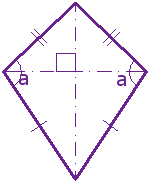Hey, it looks like a kite. It has two pairs of sides. Each pair is made up of adjacent sides that are equal in length. The angles are equal where the pairs meet. Diagonals (dashed lines) meet at a right angle, and one of the diagonal bisects (cuts equally in half) the other.

... and that's it for the special quadrilaterals.

The only regular quadrilateral is a square. So all other quadrilaterals are irregular.

## The "Family Tree" Chart

### Example: a square is also a rectangle.

So we include a square in the definition of a rectangle.

(We don't say "Having all 90° angles makes it a rectangle except when all sides are equal then it is a square.")

This may seem odd, as in daily life we think of a square as not being a rectangle ... but in mathematics it is.

Using the chart below you can answer such questions as:

• Is a Square a type of Rectangle? (Yes)
• Is a Rectangle a type of Kite? (No)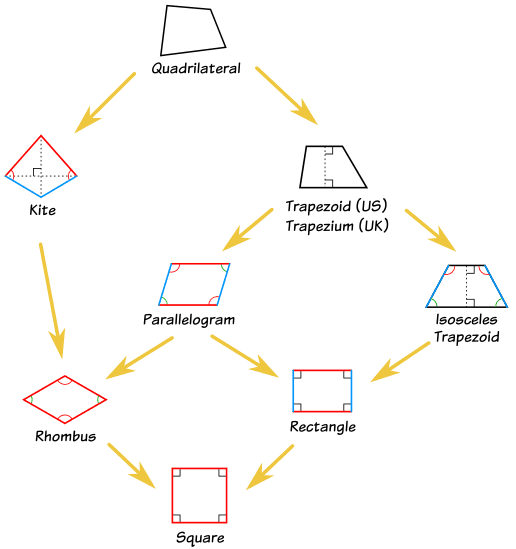Oh Yes! when two sides cross over, you call it a "Complex" or "Self-Intersecting" quadrilateral like these: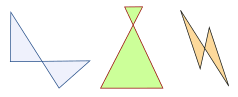They still have 4 sides, but two sides cross over.

## Polygon

A quadrilateral is a polygon. In fact it is a 4-sided polygon, just like a triangle is a 3-sided polygon, a pentagon is a 5-sided polygon, and so on.

## Play with Them

Now that you know the different types, you can play with the Interactive Quadrilaterals.

## Other Names

A quadrilateral can sometimes be called:

• Quadrangle ("four angles"), so it sounds like "triangle"
• a Tetragon ("four and polygon"), so it sounds like "pentagon", "hexagon", etc.